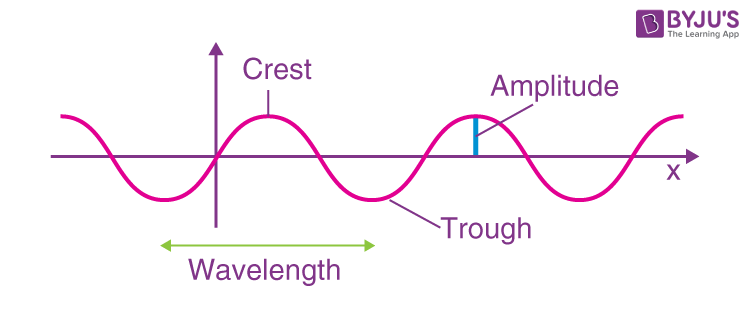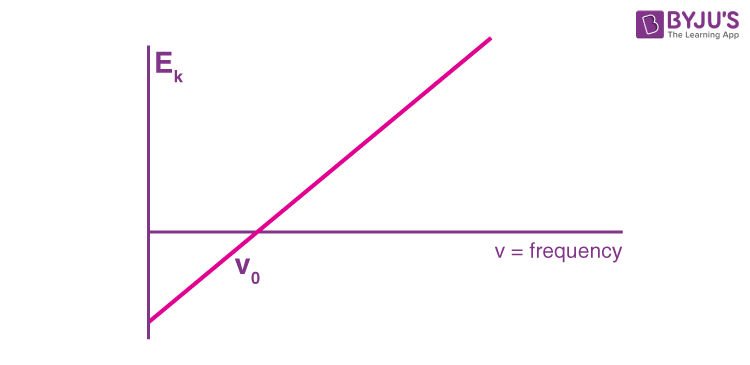# Wave Particle Duality

## What is Wave-Particle Duality?

We have heard about the nature of light and the characters it displays. Interference, reflection, refraction and diffraction are some of the characteristics. Wave-Particle Duality helps us to understand the particle and wave nature of light.

Based on the idea that light and all other electromagnetic radiation may be considered a particle or a wave nature, in 1923 physicists Louis De Broglie suggested that the same kind of duality must apply to the matter. He proposed that any particle of matter having momentum (p) Has an associated wavelength (λ).

De Broglie Wavelength formula is given by λ= h/p

Where, h is the Planck constant

for a particle of momentum mv, the wavelength is given by λ=h/mv

This equation is also applicable for photons too.

Early in this century, De Broglie began to realize the extent to which both models describe the same phenomena. Waves can be described as particles; particles, as waves. Diffraction and interference phenomena reveal a wave-like character in light. Still, the photoelectric effect, absorption and emission by atoms show light to have a particle-like character as well. Electrons have mass but can be diffracted like caves. Nature reveals a particle duality and ambiguity uncharacteristic of science. While the meaning of this wave-particle duality remains a subject of intense debate, many physicists now accept the Bohr complement-yarn principle. The two models exclude one another, yet both are necessary for a complete description of nature.According to Planck’s Hypothesis of the Quantum Theory, the energy is emitted in quanta, which are little packets of energy. He states that energy emitted is related to the frequency of the emitted light, and this can be considered a Wave-Particle Duality definition. According to Planck’s hypothesis, the quantum energy is related to the frequency by the equation E = hν.

Observing a light is one of the easiest ways to prove the duality between a particle and a wave. Since light is similar to waves, it can diffract, refract, interface, etc.

Albert Einstein’s theory of photoelectric effect significantly contributed to De Broglie’s Theory and acted as proof that particles and waves could overlap. Light can also be observed in the form of a particle called a photon. When light is seen on certain objects, electrons are released. A certain amount of energy is needed to eliminate an electron from the surface of an object. So, an electron will be emitted when a photon of greater energy than an electron hits a solid.

When the electrons are emitted, they also release kinetic energy. According to classical wave theory, the greater the intensity, the greater the energy. Because the energy of a wave is directly proportional to its amplitude, it was complex for scientists to find high-intensity lights that did not affect its overall kinetic energy.

Researchers discovered that light frequency effectively changed the amount of kinetic energy. Since some objects do not emit electrons at particular frequencies, a threshold value V0 is applied. This threshold is used to describe the amount of kinetic energy required for a photon to eject an electron. The scientists arrived at a linear relationship between frequency and kinetic energy, which can be shown by the rough sketch below.The slope of this line is known as Planck’s Constant, h = 6.63 x 10-34

Since the energy of waves and the energy of light do not coincide, we can say that light is a particle that has the property of waves.

## Frequently Asked Questions – FAQs

Q1

### State the De Broglie Wavelength formula

De Broglie Wavelength formula is given by λ= h/p.

Q2

### What is wave-particle duality?

It is the main concept of quantum mechanics, which explains how light behaves as particles and waves.

Q3

Albert Einstein
Q4

### List the process which proves the wave nature of light?

Photoelectric effect, absorption and emission by atoms.
Q5

### Which process describes the particle nature of light?

Diffraction and interference